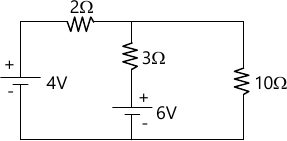MORE IN Basic Electrical Engineering (BEE)
SPPU First Year Engineering (Semester 1)
Basic Electrical Engineering (BEE)
December 2014
Total marks: --
Total time: --
INSTRUCTIONS
(1) Assume appropriate data and state your reasons
(2) Marks are given to the right of every question
(3) Draw neat diagrams wherever necessary

Answer any one question from Q1 and Q2
1 (a) Define temperature coefficient of resistance. State its unit and the factors on which it depends.
6 M
1 (b) Compare electric and magnetic circuit i.e. explain similarities and dissimilarities between them.
6 M

2 (a) A motor pump set lifts 1200 m3 of water to a height of 15 meter per hour. If the efficiency of motor is 80% and that of pump is 75%, calculate the monthly bill of set if it is used 4 hrs per day for 30 days at a rate of Rs. 5/unit.
6 M
2 (b) An iron ring with mean circumference of 140 cm and cross section of 12 cm2 is wound with 500 turns of wire. What is the relative permeability of the iron if exciting current of 2 Amp flowing in coil, produces flux of 1.2 nWb ?
6 M

Answer any one question from Q3 and Q4
3 (a) Derive the expression for energy stored in the capacitor.
6 M
3 (b) Obtain the equation for root mean square value of alternating sinusoidal current in teRMS of its peak value.
6 M

4 (a) The equation for the alternating current is given by i=100 sin 100 πt. Find the time taken by current to reach
i) 30 Amp and ii) 50 Amp for 1st time.
6 M
4 (b) A 600 KVA transformer has iron losses of 4 kW and half load copper losses are 2 kW. Calculate the efficiency of transformer at
i) half load 0.8 p.f. lagging and
ii) full load 0.8 p.f. lagging.
6 M

Answer any one question from Q5 and Q6
5 (a) Explain advantages of three phase system over single phase system.
6 M
5 (b) Sketch the voltage, current and power wavefoRMS for R-L series circuit. State the equations of v, I and P.
7 M

6 (a) A series circuit, consist of resistance of 10 ohm and inductance of 0.1 Henry, connected across one phase 50 Hz A.C. supply. If the voltage across resistance is 50 volt. Calculate
i) Voltage drop across inductance and
ii) Supply voltage.
7 M
6 (b) A coil having resistance of 50 ohm and inductance of 0.02 H is connected in parallel with a capacitor of 35 μF across a single phase 200 V, 50 Hz supply. Calculate branch currents and total current drawn by the circuit.
6 M

Answer any one question from Q1 and Q2
7 (a) State and explain Kirchhoff's laws
6 M
7 (b) Derive the expressions/formulae to convert Delta connected network into its equivalent star connected network.
7 M

8 (a) Apply Thevenin's theorem, to calculate current flowing in 10 ohm resistance, for the circuit shown in fig. 1.7 M
8 (b) Apply Superposition Theorem, to the circuit shown in Fig. 1 to calculate current flowing in 10Ω resistance.
6 M

More question papers from Basic Electrical Engineering (BEE)